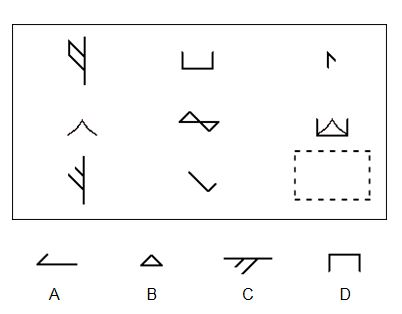# iq test

## Imagination is more important than knowledge.Imagination is more important than knowledge. For knowledge is limited to all we now know and understand, while imagination embraces the entire world, and all there ever will be to know and understand.” -Albert Einstein

IQ of famous people:

#Albert Einstein at the age of 12 – 160
#Bill Gates, founder of Microsoft – 160
#The great physicist, Stephen Hawking – 160

IQ Test Questions

IQ questions would be found on IQ tests. These questions are intended to assess a variety of mental abilities and skills, and therefore cover a wide range of different types of intelligence. Below are some general examples of the types of questions that might be found on an IQ test:

• Analogies (mathematical and verbal)
• Pattern driven (spatial and mathematical)
• Classification
• Visual
• Spatial
• Logical

While those are the general areas that an IQ test might examine, it is useful to see more specific questions. Here are a few test questions that could be encountered on an IQ test.

Sample IQ Test Questions
Verbal

1. Rearrange the following letters to make a word and choose the category in which it fits.

RAPETEKA

A. city
B. fruit
C. bird
D. vegetable

Correct answer: bird (parakeet)

Mathematical

1. Which number should come next in this series?

25,24,22,19,15

Explanation: The pattern decreases progressively: -1, -2, -3, -4, -5

Spatial

1. Which diagram results from folding the diagram on the left?Which diagram results from folding the diagram on the left?

Visualization

1.  Pick the piece that’s missing from the diagram belowPick the piece that’s missing from the diagram below

Classification

2. Which number does not belong?

 4 32 144 17 28 122 18 64 188 322 14 202

Explanation: 17 is the only odd number.

Logic

4. Which number should come next in the series

1, 3, 6, 10, 15,

A. 8
B. 11
C. 24
D. 21
E. 27

Pattern recognition

2. Find the picture that follows logically from the diagrams to the right.Find the picture that follows logically from the diagrams to the right.

• Which number should come next in the pattern?

37, 34, 31, 28

Answer: 25, the numbers are decreasing by 3

• Find the answer that best completes the analogy:

Book is to Reading as Fork is to:

1. drawing
2. writing
3. stirring
4. eating

• Find two words, one from each group, that are the closest in meaning:

Group A

talkative, job, ecstatic

Group B

angry, wind, loquacious

1. talkative and wind
2. job and angry
3. talkative and loquacious
4. ecstatic and angry

Answer: c. Talkative and Loquacious

• Which of the following can be arranged into a 5-letter English word?
1. H R G S T
2. R I L S A
3. T O O M T
4. W Q R G S

Answer: b. rails and c. motto

• What number best completes the analogy:

8:4 as 10:

1. 3
2. 7

c.24

d.5

The answer is 5 because 4 is half of 8, and 5 is half of 10.

^